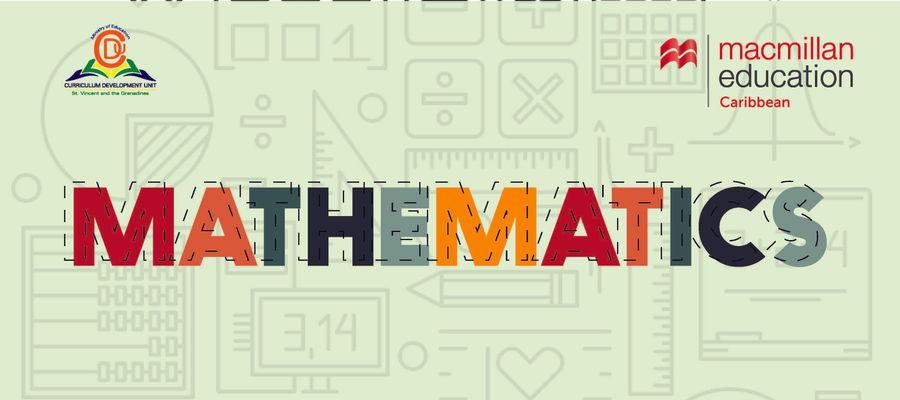# CPEA MOCK MATHEMATICS MCQ 6### Quiz Questions

• 1.
Complete the sequence 36, 48, ___, ___, 84
• A.
54, 60
• B.
60, 72
• C.
60, 62
• 2.
The combined height of Jess and Eric is 9m. If Jess is twice as tall as Eric. How tall is Eric?
• A.
18m
• B.
4.5m
• C.
3m
• 3.
Calculate the volume of cube whose height is 2cm
• A.
8cm³
• B.
6cm³
• C.
8cm²
• 4.
In a school there is 1 teacher for every 25 students. If there are 725 students. How many teachers are in the school?
• A.
750
• B.
29
• C.
18125
• 5.
What is the small number you can get from the digits: 7 1 4 3
• A.
1347
• B.
1437
• C.
7431
• 6.
If 3/4 of a number is 72. What is the number?
• A.
54
• B.
84
• C.
96
• 7.
Calculate the area of a triangle whose base is 6cm and height is 15cm
• A.
42cm²
• B.
90cm²
• C.
45cm²
• 8.
What is the product of 3.36 and 1.4 to the nearest whole number
• A.
4.704
• B.
5
• C.
4
• 9.
Three rotis cost \$12.30. What is the cost of 5 rotis?
• A.
\$2.46
• B.
\$61.50
• C.
\$20.50
• 10.
Which of the following units is most appropriate  for measuring the weight of the pencil?
• A.
g
• B.
kg
• C.
tonnes

• 1.
Result 1